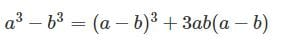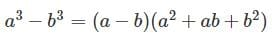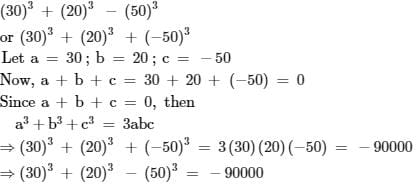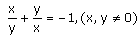Test: Algebraic Identities Cubic Type

# Test: Algebraic Identities Cubic Type

Test Description

## 20 Questions MCQ Test Mathematics (Maths) Class 9 | Test: Algebraic Identities Cubic Type

Test: Algebraic Identities Cubic Type for Class 9 2023 is part of Mathematics (Maths) Class 9 preparation. The Test: Algebraic Identities Cubic Type questions and answers have been prepared according to the Class 9 exam syllabus.The Test: Algebraic Identities Cubic Type MCQs are made for Class 9 2023 Exam. Find important definitions, questions, notes, meanings, examples, exercises, MCQs and online tests for Test: Algebraic Identities Cubic Type below.
Solutions of Test: Algebraic Identities Cubic Type questions in English are available as part of our Mathematics (Maths) Class 9 for Class 9 & Test: Algebraic Identities Cubic Type solutions in Hindi for Mathematics (Maths) Class 9 course. Download more important topics, notes, lectures and mock test series for Class 9 Exam by signing up for free. Attempt Test: Algebraic Identities Cubic Type | 20 questions in 20 minutes | Mock test for Class 9 preparation | Free important questions MCQ to study Mathematics (Maths) Class 9 for Class 9 Exam | Download free PDF with solutions
 1 Crore+ students have signed up on EduRev. Have you?
Test: Algebraic Identities Cubic Type - Question 1

### Expansion and simplification of 8(3h - 4) + 5(h - 2) gives

Detailed Solution for Test: Algebraic Identities Cubic Type - Question 1

Now, 8 (3h - 4) + 5 (h - 2)

= 24h - 32 + 5h - 10, the expansion

= 24h + 5h - 32 - 10

= 29h - 42, the simplification

Test: Algebraic Identities Cubic Type - Question 2

### Evaluate (11)3​

Detailed Solution for Test: Algebraic Identities Cubic Type - Question 2

11³= (10+1)³=1000+1+30(11)=1001+330=1331

Test: Algebraic Identities Cubic Type - Question 3

### Factorise:(3x- 5y)3+ (5y – 2z)3 + (2z – 3x)3

Detailed Solution for Test: Algebraic Identities Cubic Type - Question 3

We don't have to cube them all to factorise. Now, One thing should be remembered that, in time of this type of factorisation we have to first add the polynomials to check if the sum is zero or not.If it is zero, then the factorisation will be in form of 3(abc). Now, lets sum them, (3x-5y) +(5y-2z) +(2z-3x).The answer is zero. So put the expression in form of 3(abc). So the factorisation of the expression is 3(3x-5y)(5y-2z)(2z-3x).

Test: Algebraic Identities Cubic Type - Question 4

Evaluate: 53 – 23 – 33

Detailed Solution for Test: Algebraic Identities Cubic Type - Question 4

53 - 23 - 33​​​​​​​
= 125 - 8 - 27
= 90

Test: Algebraic Identities Cubic Type - Question 5

Factorise : 8a3+ b3 + 12a2b + 6a b2

Detailed Solution for Test: Algebraic Identities Cubic Type - Question 5

8a3 + b3+ 12a2b + 6ab2
8a3 + b3+ 12a2b + 6ab2
= (2a)3 + (b)3 + 3(2a)(b) (2a + b)
= (2a + b)3 | Using Identity VI
= (2a + b)(2a + b)(2a + b)

Test: Algebraic Identities Cubic Type - Question 6

Evaluate (x+y+z)2

Test: Algebraic Identities Cubic Type - Question 7

Factorise : 8a3 + b3 + 12a2 b + 6ab2

Detailed Solution for Test: Algebraic Identities Cubic Type - Question 7

8a3 + b3+ 12a2b + 6ab2
8a3 + b3+ 12a2b + 6ab2
= (2a)3 + (b)3 + 3(2a)(b) (2a + b)
= (2a + b)3 | Using Identity VI
= (2a + b)(2a + b)(2a + b)

Test: Algebraic Identities Cubic Type - Question 8

p3+ q3 + r3 – 3pqr = ?​

Test: Algebraic Identities Cubic Type - Question 9

Factorize: 125a3 – 27b3 – 225a2b + 135ab2.​

Test: Algebraic Identities Cubic Type - Question 10

Evaluate (104)3

Detailed Solution for Test: Algebraic Identities Cubic Type - Question 10

(104)³
=(100 + 4)³
Use ( a +b)³ = a³ + b³ + 3ab( a + b)
then,
( 100 + 4)³ = ( 100)³ +(4)³ +3(100)(4)(100+4)
=(10²)³ + 64 + 1200(104)
=1000000 + 64 + 124800
=1124864.

Test: Algebraic Identities Cubic Type - Question 11

Factorize: (x – y)3 + (y – z)3 + (z – x)3

Test: Algebraic Identities Cubic Type - Question 12

Factorise: x7y + xy7

Test: Algebraic Identities Cubic Type - Question 13

What is the value 83 – 33 (without solving the cubes)?​

Detailed Solution for Test: Algebraic Identities Cubic Type - Question 13

(8-3)((8)² + 8×3 + (3)²) = 5(64 + 24 + 9) = 5×97 = 485

Test: Algebraic Identities Cubic Type - Question 14

p3+ q3 = ?​

Test: Algebraic Identities Cubic Type - Question 15

What is the value of 53 – 13(without solving cube)?

Detailed Solution for Test: Algebraic Identities Cubic Type - Question 15

53 - 13 can be solved using the identity ;

(a3 - b3) = (a - b) (a2 + b2 + ab)

53 - 13 = ( 5 - 1 ) ( 52 + 12 + 5*1)

=(4) (25 + 1 + 5)

= (4) (31)

= 124

Test: Algebraic Identities Cubic Type - Question 16

Factorize: 27x3 – 125y3

Test: Algebraic Identities Cubic Type - Question 17

a3 – b3 equals​

Detailed Solution for Test: Algebraic Identities Cubic Type - Question 17

It is the identity equationIn the fraction equationTest: Algebraic Identities Cubic Type - Question 18

Evaluate: 303 + 203 – 503.​

Detailed Solution for Test: Algebraic Identities Cubic Type - Question 18

We have to find the value ofTest: Algebraic Identities Cubic Type - Question 19

If, the value of x3 – y3 is :

Test: Algebraic Identities Cubic Type - Question 20

Factorization of 103²-9 yields

## Mathematics (Maths) Class 9

88 videos|397 docs|109 tests
 Use Code STAYHOME200 and get INR 200 additional OFF Use Coupon Code
Information about Test: Algebraic Identities Cubic Type Page
In this test you can find the Exam questions for Test: Algebraic Identities Cubic Type solved & explained in the simplest way possible. Besides giving Questions and answers for Test: Algebraic Identities Cubic Type , EduRev gives you an ample number of Online tests for practice

## Mathematics (Maths) Class 9

88 videos|397 docs|109 tests